# Exponents in Math: Tutoring Solution Chapter Exam

Exam Instructions:

Choose your answers to the questions and click 'Next' to see the next set of questions. You can skip questions if you would like and come back to them later with the yellow "Go To First Skipped Question" button. When you have completed the practice exam, a green submit button will appear. Click it to see your results. Good luck!

### Page 1

#### Question 1 1. Which of the following is equivalent to the expression below?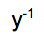#### Question 2 2. Simplify the expression below using only positive exponents.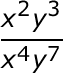#### Question 3 3. Using positive exponents, simplify the expression.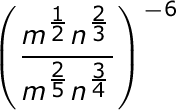#### Question 4 4. Simplify using ONLY positive exponents.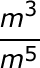#### Question 5 5. Simplify the following expression using positive exponents.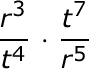### Page 2

#### Question 6 6. Simplify using only positive exponents.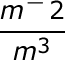#### Question 7 7. Which of the following is equivalent to the following expression?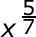#### Question 8 8. Which of the following radical expressions is equal to this exponential expression?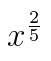#### Question 9 9. Simplify the expression below.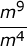#### Question 10 10. Simplify the following expression using positive exponents only.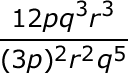### Page 3

#### Question 11 11. Simplify the following expression using positive exponents.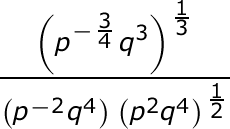#### Question 12 12. Which of the following exponential expressions is equal to this radical expression?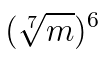#### Question 13 13. Simplify the expression using positive exponents.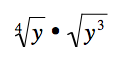#### Question 15 15. Which of the following is equivalent to the expression below?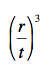### Page 4

#### Question 16 16. Which of the following would be true regarding the following exponential expression?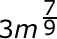#### Question 17 17. Simplify the expression below using positive exponents only.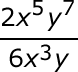#### Question 18 18. Simplify the following expression.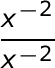#### Question 19 19. Which of the following is equivalent to the expression below?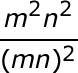#### Question 20 20. Simplify the following expression.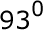### Page 5

#### Question 21 21. Simplify using ONLY positive exponents.#### Question 22 22. Which of the following is equivalent to the following expression?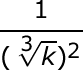#### Question 23 23. Simplify the expression below using positive exponents.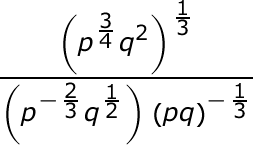#### Question 24 24. Simplify this expression using positive exponents.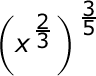#### Question 25 25. Which of the following is equivalent to the expression below?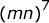#### Exponents in Math: Tutoring Solution Chapter Exam Instructions

Choose your answers to the questions and click 'Next' to see the next set of questions. You can skip questions if you would like and come back to them later with the yellow "Go To First Skipped Question" button. When you have completed the practice exam, a green submit button will appear. Click it to see your results. Good luck!

Support